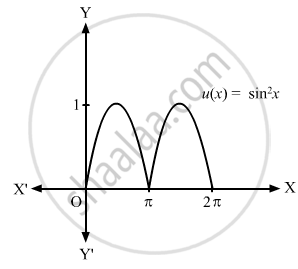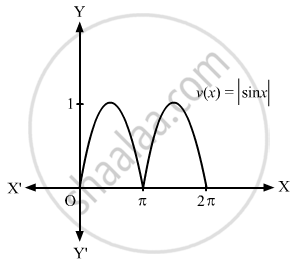Advertisement Remove all ads

# Sketch the Graph of the Following Function: U ( X ) = Sin 2 X , 0 ≤ X ≤ 2 π V ( X ) = | Sin X | , 0 ≤ X ≤ 2 π - Mathematics

Short Note

Sketch the graph of the following function:

$u\left( x \right) = \sin^2 x, 0 \leq x \leq 2\pi v\left( x \right) = \left| \sin x \right|, 0 \leq x \leq 2\pi$

Advertisement Remove all ads

#### Solution

$u\left( x \right) = \sin^2 x, 0 \leq x \leq 2\pi$
$v\left( x \right) = \left| \sin x \right|, 0 \leq x \leq 2\pi$

 x 0 $\pi$ $u\left( x \right) = \sin^2 x$ 0 0x 0 $\pi$ $v\left( x \right) = \left| \sin x \right|$ 0 0Concept: Graphs of Trigonometric Functions
Is there an error in this question or solution?
Advertisement Remove all ads

#### APPEARS IN

RD Sharma Class 11 Mathematics Textbook
Chapter 6 Graphs of Trigonometric Functions
Exercise 6.1 | Q 1.7 | Page 5
Advertisement Remove all ads
Advertisement Remove all ads
Share
Notifications

View all notifications

Forgot password?